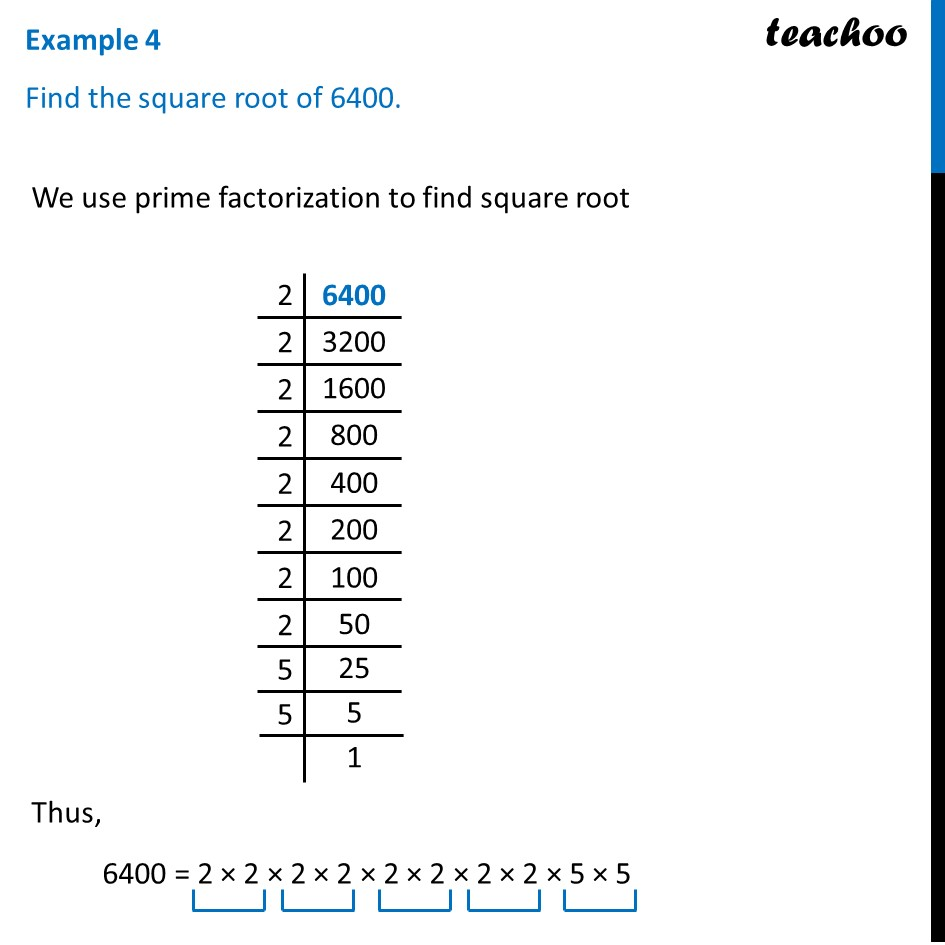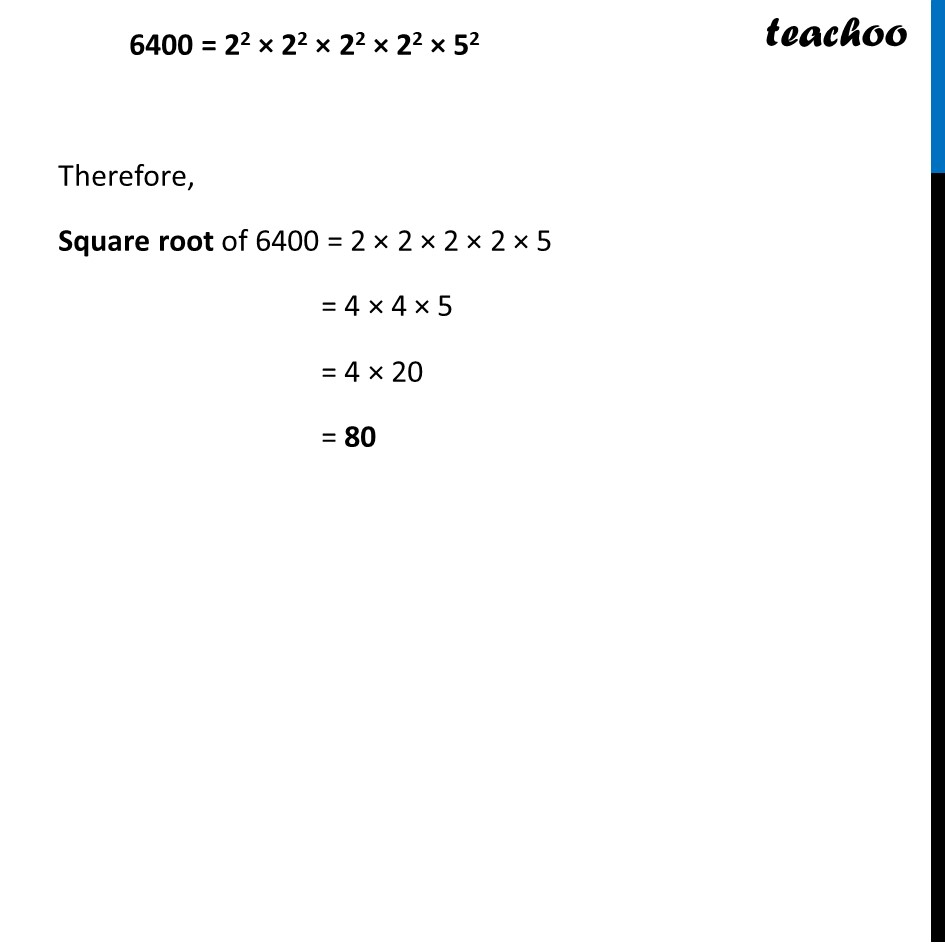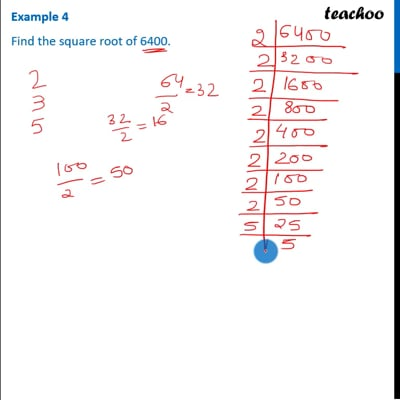Examples

Chapter 6 Class 8 Squares and Square Roots
Serial order wiseThis video is only available for Teachoo black users

### Transcript

Example 4 Find the square root of 6400. We use prime factorization to find square root We use prime factorization to find square root Thus, 6400 = 2 × 2 × 2 × 2 × 2 × 2 × 2 × 2 × 5 × 5 6400 = 22 × 22 × 22 × 22 × 52 Therefore, Square root of 6400 = 2 × 2 × 2 × 2 × 5 = 4 × 4 × 5 = 4 × 20 = 80